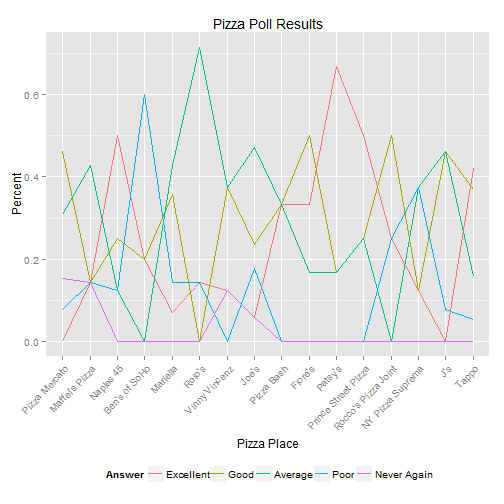For a d3 bar plot visit https://www.jaredlander.com/plots/PizzaPollPlot.html.

I finally compiled the data from all the pizza polling I’ve been doing at the New York R meetups. The data are available as json at https://www.jaredlander.com/data/PizzaPollData.php.

This is easy enough to plot in R using ggplot2.

require(rjson)
require(plyr)
pizzaJson <- fromJSON(file = "http://jaredlander.com/data/PizzaPollData.php")
pizza <- ldply(pizzaJson, as.data.frame)

##   polla_qid      Answer Votes pollq_id                Question
## 1         2   Excellent     0        2  How was Pizza Mercato?
## 2         2        Good     6        2  How was Pizza Mercato?
## 3         2     Average     4        2  How was Pizza Mercato?
## 4         2        Poor     1        2  How was Pizza Mercato?
## 5         2 Never Again     2        2  How was Pizza Mercato?
## 6         3   Excellent     1        3 How was Maffei's Pizza?
## 1  Pizza Mercato 1.344e+09         13  0.0000
## 2  Pizza Mercato 1.344e+09         13  0.4615
## 3  Pizza Mercato 1.344e+09         13  0.3077
## 4  Pizza Mercato 1.344e+09         13  0.0769
## 5  Pizza Mercato 1.344e+09         13  0.1538
## 6 Maffei's Pizza 1.348e+09          7  0.1429

require(ggplot2)
ggplot(pizza, aes(x = Place, y = Percent, group = Answer, color = Answer)) +
geom_line() + theme(axis.text.x = element_text(angle = 46, hjust = 1), legend.position = "bottom") +
labs(x = "Pizza Place", title = "Pizza Poll Results")But given this is live data that will change as more polls are added I thought it best to use a plot that automatically updates and is interactive. So this gave me my first chance to need rCharts by Ramnath Vaidyanathan as seen at October’s meetup.

require(rCharts)
pizzaPlot <- nPlot(Percent ~ Place, data = pizza, type = "multiBarChart", group = "Answer")
pizzaPlot$xAxis(axisLabel = "Pizza Place", rotateLabels = -45) pizzaPlot$yAxis(axisLabel = "Percent")
pizzaPlot$chart(reduceXTicks = FALSE) pizzaPlot$print("chart1", include_assets = TRUE)


Unfortunately I cannot figure out how to insert this in WordPress so please see the chart at https://www.jaredlander.com/plots/PizzaPollPlot.html. Or see the badly sized one below.

There are still a lot of things I am learning, including how to use a categorical x-axis natively on linecharts and inserting chart titles. I found a workaround for the categorical x-axis by using tickFormat but that is not pretty. I also would like to find a way to quickly switch between a line chart and a bar chart. Fitting more labels onto the x-axis or perhaps adding a scroll bar would be nice too.Jared Lander is the Chief Data Scientist of Lander Analytics a New York data science firm, Adjunct Professor at Columbia University, Organizer of the New York Open Statistical Programming meetup and the New York and Washington DC R Conferences and author of R for Everyone.